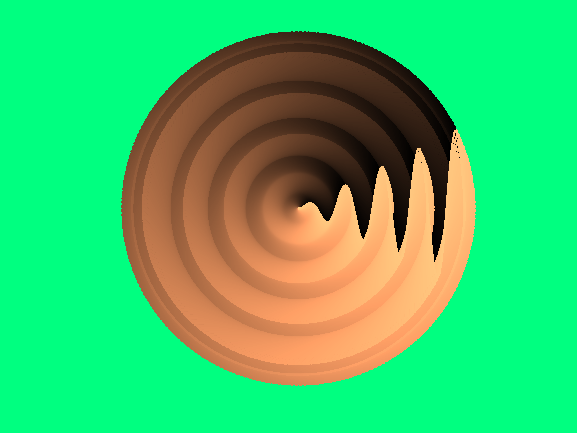• /
• # Cymbal Wave

on 11 Oct 2021
• 11
• 23
• 0
• 0
• 276
% This is a demo created by MathWorks community team.
% Author: Ned Gulley
% Please do not vote on this demo entry.
figure('Color','#00FF80')
x = linspace(0, 9*pi);
y = 0.5*x.*sin(x);
h1 = plot(x, y, LineWidth=1.5);
k = 2^8;
c = copper(k);
for n = 1:k
h2 = copyobj(h1, gca);
t = hgtransform;
set(h2,Parent=t,Color=c(n,:))
theta = n*2*pi/k;
rot = makehgtform(ZRotate=theta);
set(t, Matrix=rot);
end
axis equal
axis off